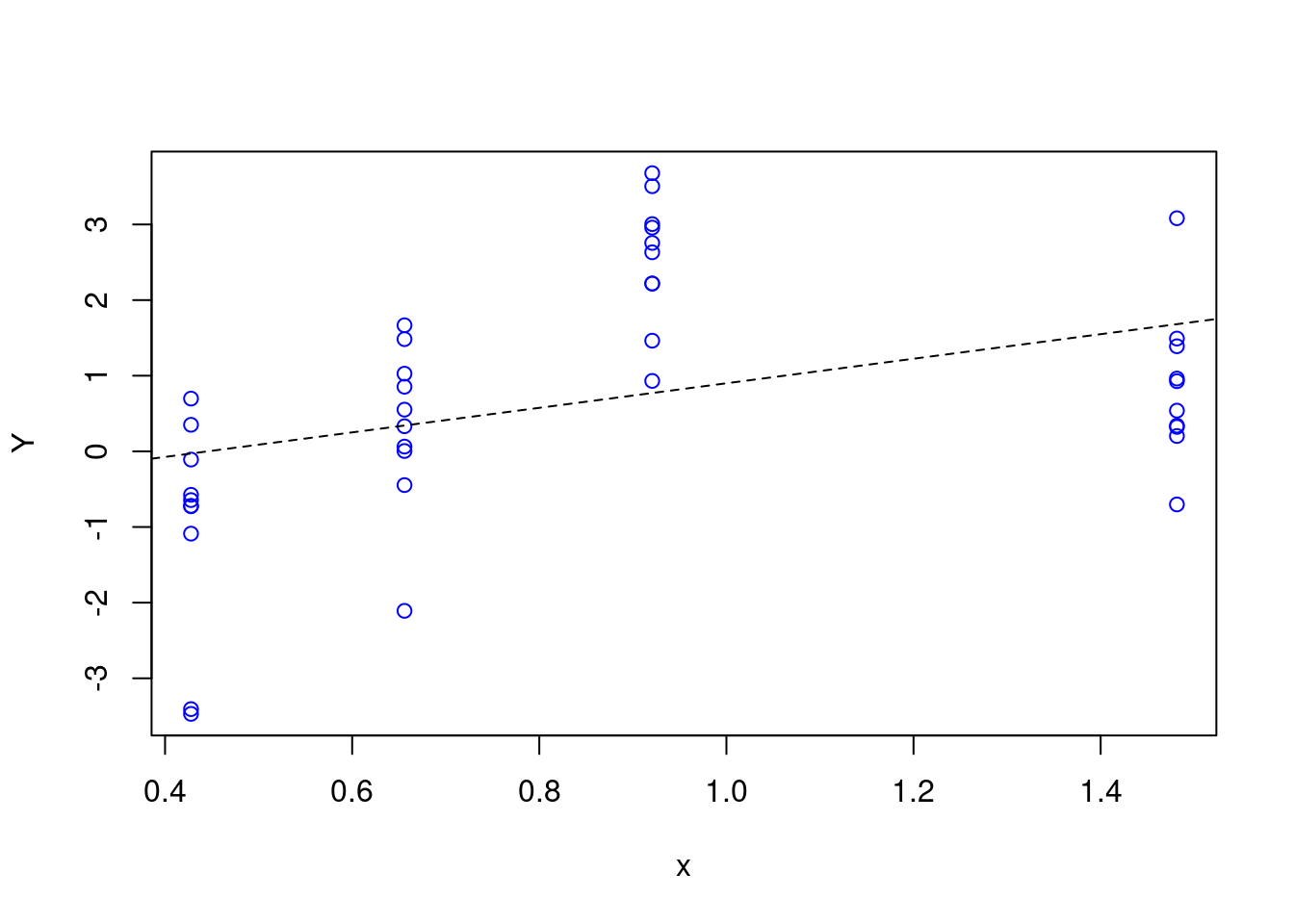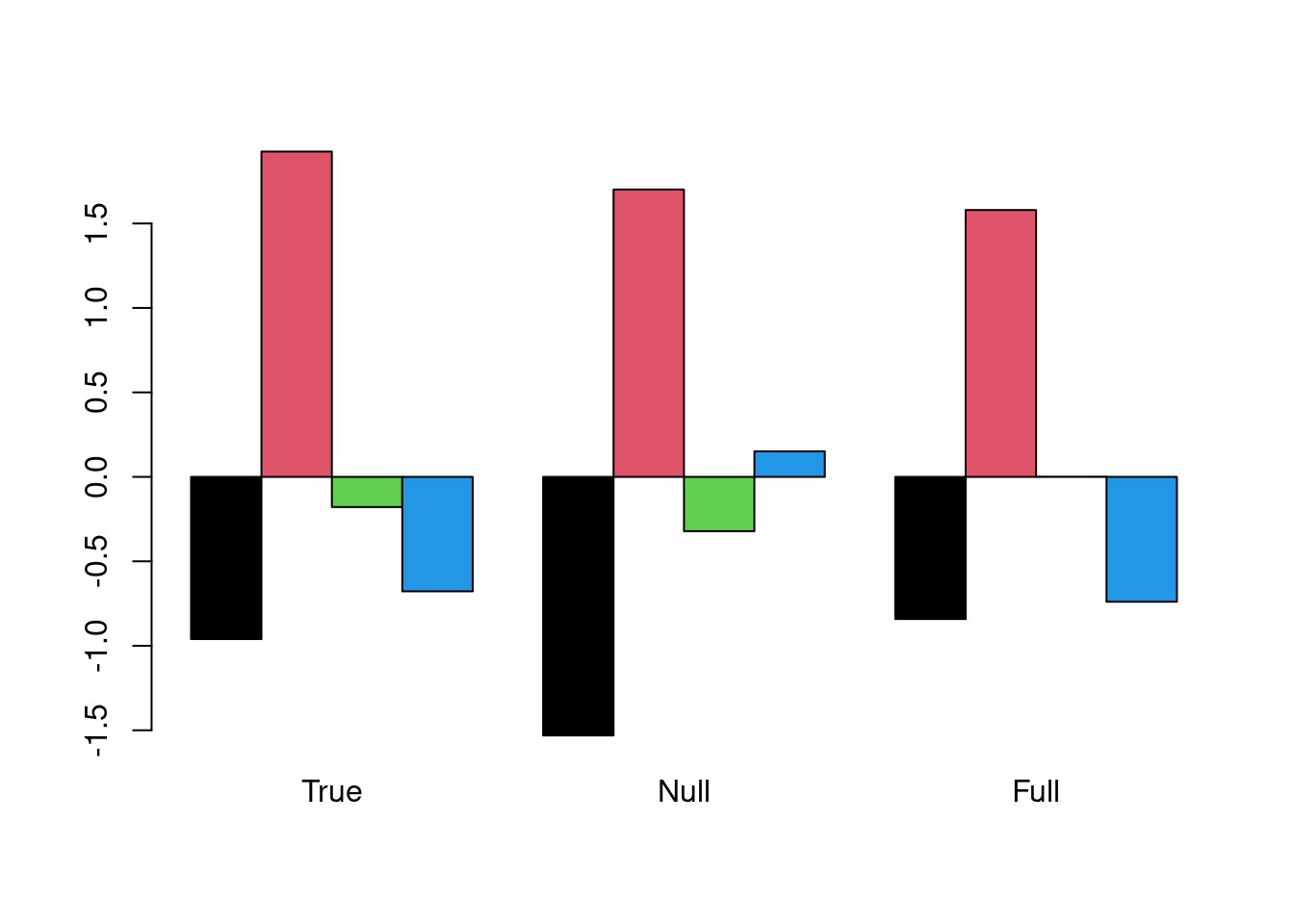# An issue with the random intercepts model

## Model

Consider a linear mixed model like

$\begin{equation} Y_{ij} = \alpha + u_i + \beta x_{ij} + \epsilon_{ij}, \quad j = 1, \ldots, n_i; \; i = 1, \ldots, K, \tag{1} \end{equation}$

where $$u_1, \ldots, u_K$$ are iid random intercepts (drawn from $$N(0, \tau^2)$$), $$x_{ij}$$ are measures on a covariate (continuous type) and $$\epsilon_{ij}$$ are iid $$N(0, \sigma^2)$$. If the $$u$$s and the $$x$$es are independent, we can write the model as

$\begin{equation} \label{eq:two} Y_{ij} = \alpha + \beta x_{ij} + \omega_{ij}, \tag{2} \end{equation}$ where the $$\omega_{ij} = u_i + \epsilon_{ij}$$ are multivariate normal with mean zero and a block diagonal covariance matrix. The least squares fit of this model yields an unbiased estimate of $$\beta$$, but the standard error estimate based on the assumption of uncorrelated errors is biased.

The issue we are interested in is if it is meaningful to fit model (1) when the $$x$$es are constant within clusters. The question is reasonable, since the corresponding fixed effects model would be overdetermined.

## The issue

We rewrite (1) slightly to get

$\begin{equation} Y_{ij} = \alpha + u_i + \beta x_{i} + \epsilon_{ij}, \quad j = 1, \ldots, n_i; \; i = 1, \ldots, K, \tag{3} \end{equation}$

where the only difference is that the $$x$$es now are constant within clusters.

It turns out that model (3) is meaningful to estimate, and one intuitive argument is that the model has four parameters to estimate $$(\alpha, \beta, \tau^2, \sigma^2)$$, while the corresponding fixed effects model contains $$K + 2$$ parameters, $$K$$ intercepts and 2 variances. The random intercepts model implies a shrinkage of the intercepts in the estimation procedure. (Note: What if $$K = 2$$?)

## Simulation

We illustrate ideas with some simulated data.There are four clusters with ten observations in each. The dashed line is the result of fitting model (2), the simple linear regression, to data. The output is


Call:
lm(formula = Y ~ x, data = dd)

Residuals:
Min      1Q  Median      3Q     Max
-3.4419 -0.7623 -0.1351  1.1878  2.9066

Coefficients:
Estimate Std. Error t value Pr(>|t|)
(Intercept)  -0.7230     0.5956  -1.214    0.232
x             1.6230     0.6230   2.605    0.013 *
---
Signif. codes:  0 '***' 0.001 '**' 0.01 '*' 0.05 '.' 0.1 ' ' 1

Residual standard error: 1.549 on 38 degrees of freedom
Multiple R-squared:  0.1515,    Adjusted R-squared:  0.1292
F-statistic: 6.787 on 1 and 38 DF,  p-value: 0.01304

If we fit a random intercepts model to data (with the function lmer in the package lme4), we get

Loading required package: Matrix
Linear mixed model fit by maximum likelihood  ['lmerMod']
Formula: Y ~ x + (1 | kommun)
Data: dd

AIC      BIC   logLik deviance df.resid
138.8    145.6    -65.4    130.8       36

Scaled residuals:
Min      1Q  Median      3Q     Max
-2.3440 -0.4089  0.1326  0.5578  1.9287

Random effects:
Groups   Name        Variance Std.Dev.
kommun   (Intercept) 1.047    1.023
Residual             1.231    1.109
Number of obs: 40, groups:  kommun, 4

Fixed effects:
Estimate Std. Error t value
(Intercept)   -0.723      1.316  -0.549
x              1.623      1.376   1.179

Correlation of Fixed Effects:
(Intr)
x -0.912

Note that we get exactly the same estimate of the regression parameter $$\beta$$ in the two analyses, but when we include information on clustering, its standard error is almost doubled, and non-significant.

(New 2020-05-19:) The fact that we got identical regression coefficient estimates under the two models seems to an artefact of the balanced design, that is, the clusters are all of the same size. Further, It will not hold (in general) for a binomial or Poisson model, even if the design is balanced.

The IntraCorrelation Coefficient (ICC) $$\rho$$ is defined as

$\begin{equation} \rho = \frac{\tau^2}{\tau^2 + \sigma^2} \end{equation}$

We find the estimated variances under Random effects in the output from lmer. We get $$\hat{\rho} = 0.460$$, which can be interpreted as the proportion of the total variance that is attributed to the clustering.

(New 2020-05-29:) From the lmer fits we can extract the predicted values of the cluster effects:Remember that this is simulated data so we know the true simulated values (“True”). “Null” stands for a model with only cluster effects, and in “Full” the covariate $$x$$ is included.

## Final remark

We have only discussed linear mixed models here. Similar conclusions should hold for binomial or Poisson models. The issue of ICC will be a tad harder to explain, though.